﻿ Calculation of a fluid bed using Discrete Particle Modelling - XSim

# Calculation of a fluid bed using Discrete Particle Modelling

Update: March 16, 2018
OpenFOAM 4.x

## Case directory

\$FOAM_TUTORIALS/lagrangian/DPMFoam/Goldschmidt

## Summary

We perform unsteady calculations of a fluid bed for 5 seconds using Discrete Particle Modelling. The computational domain is a rectangular box with air flowing in vertically from the region "bottom" at 1.875 m/s and flowing out from the region "top". The side walls are assumed to be no-slip walls. And thickness direction walls are assumed to be symmetric walls.Model geometry

The initial positions and properties of the discrete particles are defined in the files "kinematicCloudPositions" and "kinematicCloudProperties" in the directory "constant", respectively. Fluid calculation is performed without using the turbulence model.

The meshes are as follows, and the number of mesh is 54000.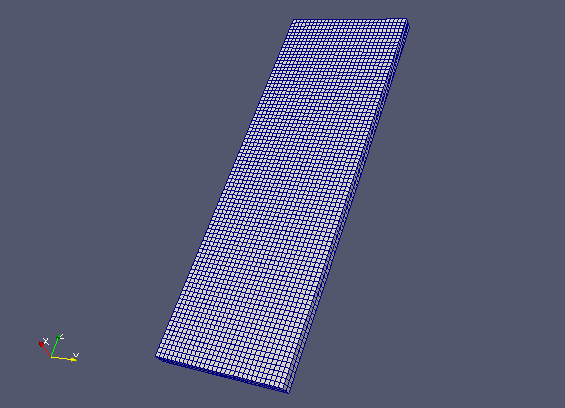Meshes

The calculation result is as follows.

Discrete particles's motion (at 1/4 speed, color represents magnitude of flow velocity)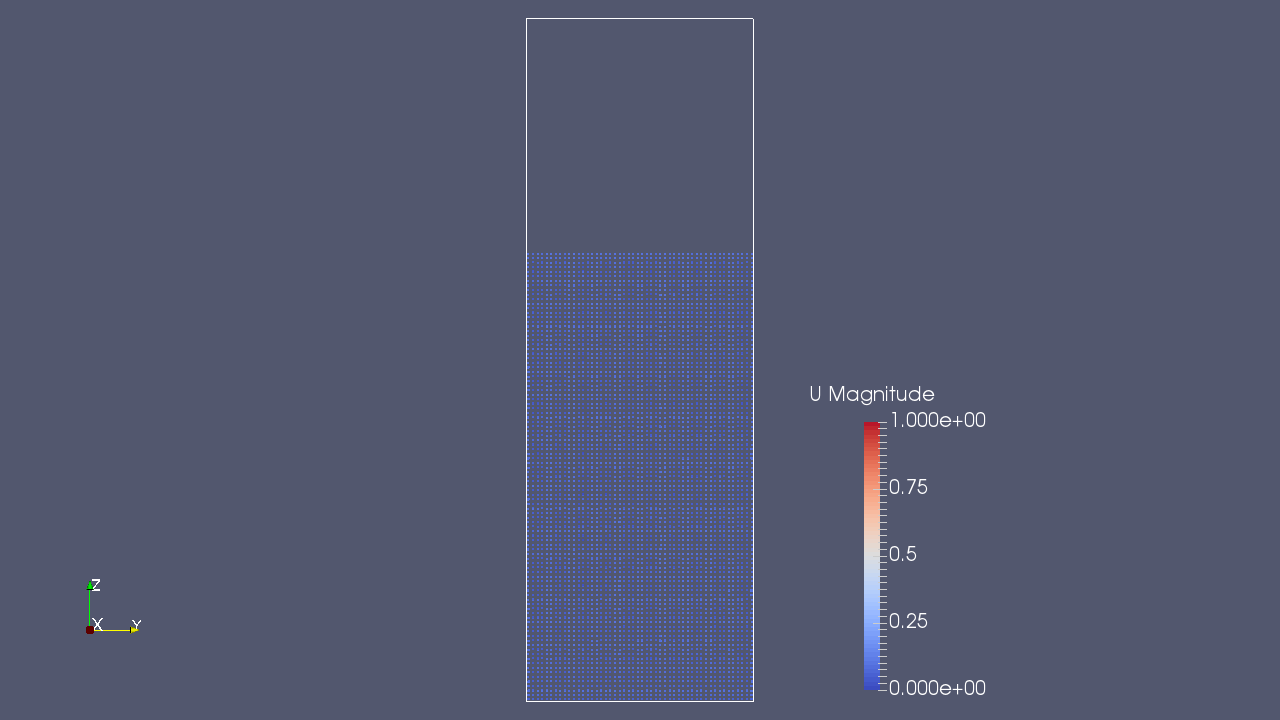The discrete particles and flow velocity at time 0 sec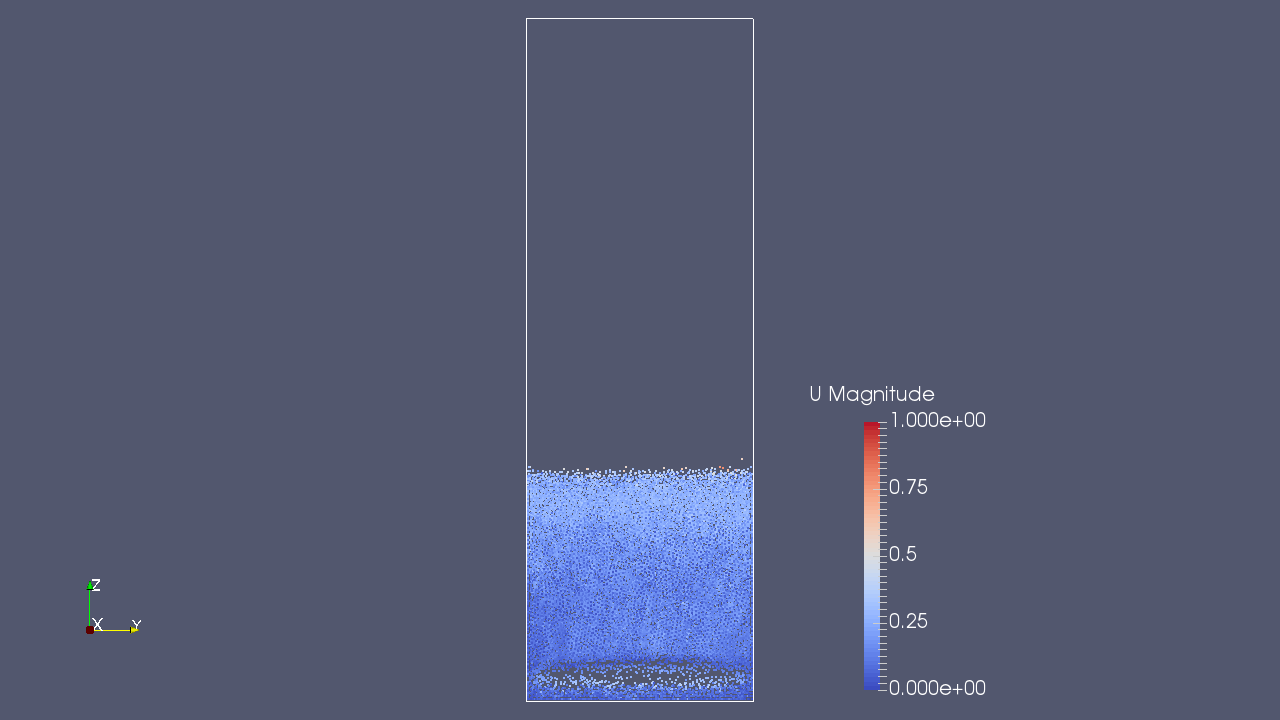The discrete particles and flow velocity at time 0.25 sec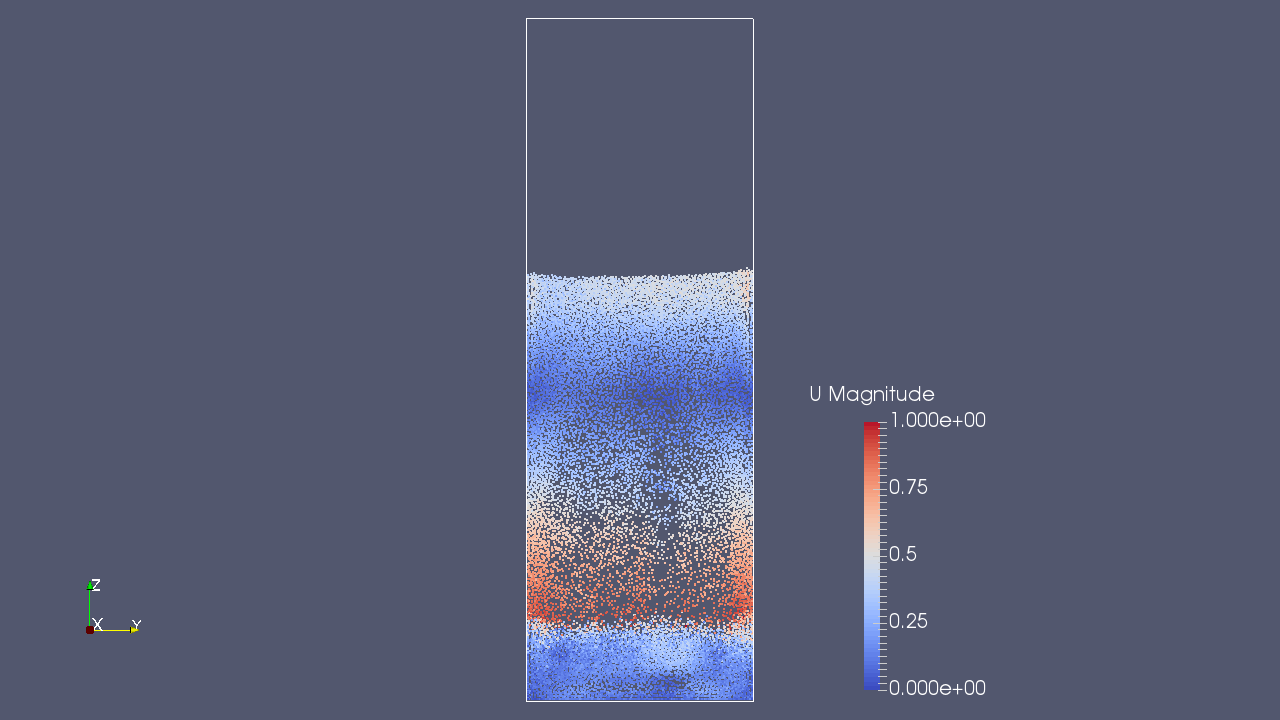The discrete particles and flow velocity at time 0.5 sec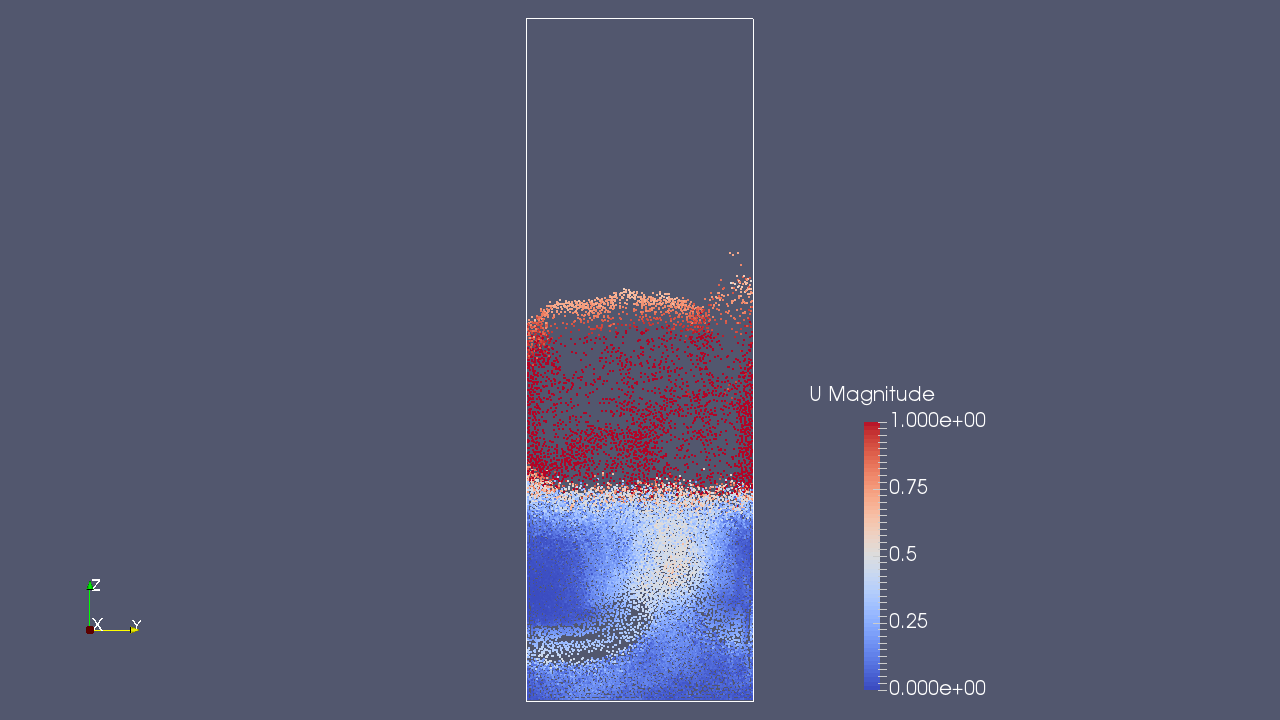The discrete particles and flow velocity at time 0.75 sec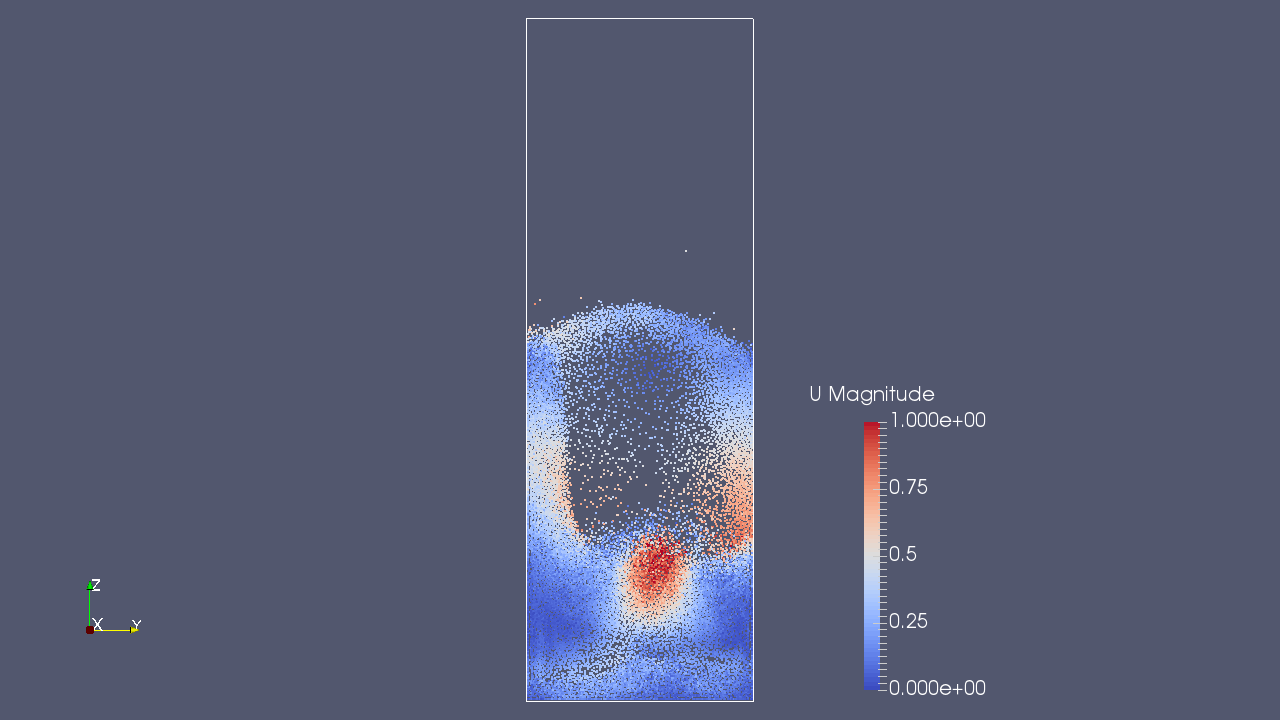The discrete particles and flow velocity at time 1 sec

## Commands

cp -r \$FOAM_TUTORIALS/lagrangian/DPMFoam/Goldschmidt Goldschmidt
cd Goldschmidt

blockMesh
decomposePar
mpirun -np 6 DPMFoam -parallel
reconstructParMesh
reconstructPar

paraFoam

## Calculation time

17 hours 38 minutes 18.6 seconds *6 parallel, Inter(R) Core(TM) i7-2600 CPU @ 3.40GHz 3.40GHz

## Reference

• "Hydrodynamic Modelling of Fluidised Bed Spray Granulation", M.J.V. Goldschmidt, Twente University Press (TUP), 2001/8/31
• "Discrete element modelling of fluidised bed spray granulation", M.J.V. Goldschmidt, G.G.C. Weijers, R. Boerefijn, J.A.M. Kuipers, 2002/7/21
• OpenFOAM 2.3.0: Discrete Particle Modelling - OpenFOAM, 2014/2/17